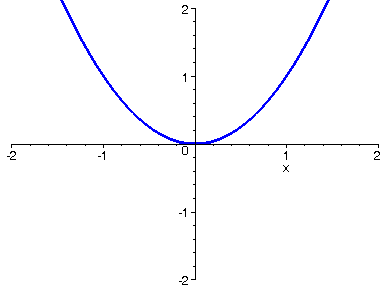THE POWER FUNCTION X TO THE NTH POWER

Years ago during the very early days of personal computers, I wrote a program in BASIC for graphing functions, and I was intrigued for the very first time by the behavior of y = x^n as x is raised to higher and higher powers. In the interval from -1 to 1 the graph flattens out more as fractions from that interval are raised to higher powers. Outside of this interval, in contrast, the magnitude of y increases greatly with each increase of the power n. All of the graphs, though, pass through the points (1, 1) and through (-1, 1) when n is even and (-1, -1) when n is odd. The animations below illustrate what happens with these graphs as we let increase the power. Enjoy!

Even Powers:

> A := seq(plot(x^(2*i),x=-2..2, numpoints=500, thickness=3, color=blue), i=1..30):

> display(A, insequence=true, view=[-2..2, -2..2]);Odd Powers:

> B := seq(plot(x^(2*i-1),x=-2..2, numpoints=500, thickness=3, color=red), i=1..30):

> display(B, insequence=true, view=[-2..2, -2..2]);>